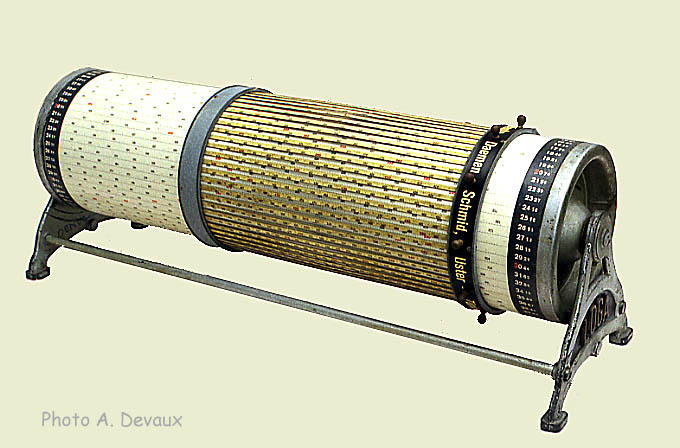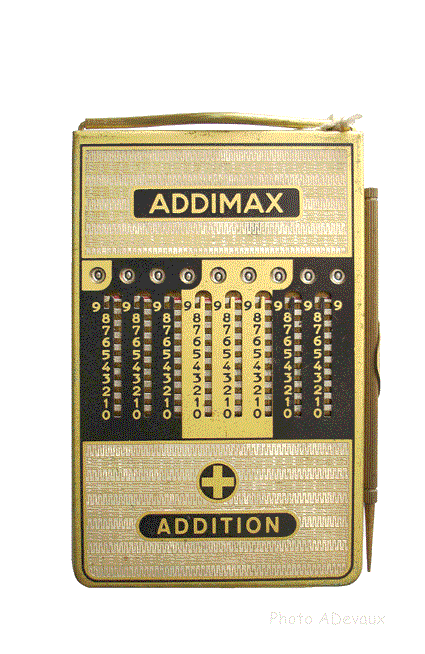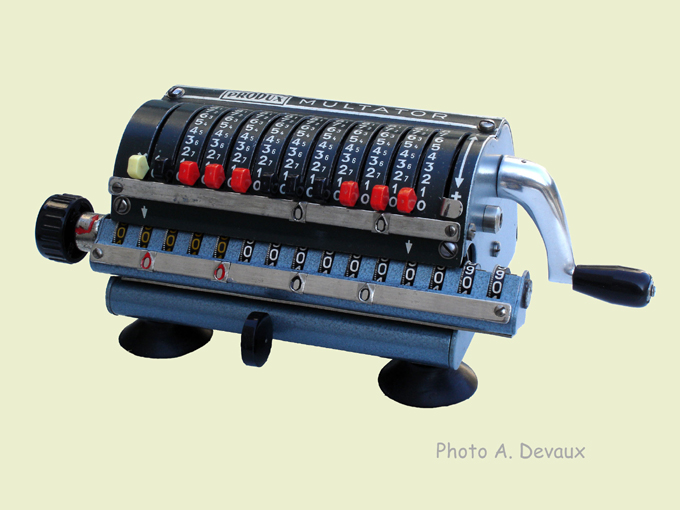Instruments of calculation and calculators

Instruments of calculations:

On the contrary of a calculating machine, an instrument of calculations requires the participation of the operator during the operation (for the carry for example). These instruments help the operator in the calculation process. I put the slide rules in this category.

Instruments giving approximate results:We find in this category the instruments for which the result of the operation is obtained by reading a number facing a reference mark. Most known of these instruments are the slide rules that one finds in various forms (linear, circular, cylindrical...). They give results on which the precision depends the skill with which the operator handles the instrument and is able to evaluate the result of the operation.

Instruments giving exact results:The apparatus of calculations giving exact results help the operator to achieve operations such as the addition, the subtraction, the multiplication and/or division (to speak only about the four fundamental operations). However, the the intervention of the operator is required during calculation in order to obtain a correct result. One find in this category: abacus, rods of Neper, rods of Genaille and Lucas as well as the instruments with cogged slide bars.

Calculating machines:A calculating machine is an apparatus which does not require the intervention of the operator with regard to the carry.

The operator introduces the numbers on which he wants to make calculation and actuates the mechanism in an adequate way to perform the desired operation.

However, the least sophisticated calculator requires the attention of the operator to count the exact number of cycles (number of revolutions of the driving shaft) and to shift of the carriage. This work is facilitate by the presence of a counter which indicates the number of cycles carried out for the various positions of the carriage.

The most sophisticated calculators deal with the totality of the operation (after input of the operator and of the operand). The only thing the operator has to do is depress the key corresponding to the operation he wants to perform.